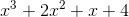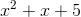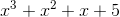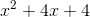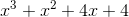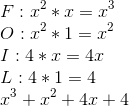## Example Questions

### Example Question #3 : Simplifying

Simplify the following binomial: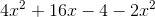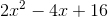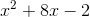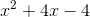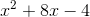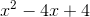Explanation:

The equation that is presented is:To get the correct answer, you first need to combine all of the like terms. So, you can subtract the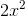from the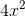, leaving you with: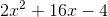From there, you can reduce the numbers by their greatest common denominator, in this case,:### Example Question #4 : Simplifying

Simplify the following binomial expression: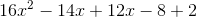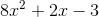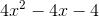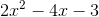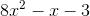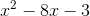Explanation:

First, combine all of the like terms that you are able: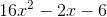Then, reduce by the greatest common denominator (in this case,):### Example Question #5 : Simplifying

Simplify the following binomial: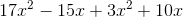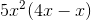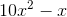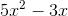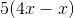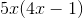Explanation:

The equation presented in the problem is:First you have to combine the like terms, i.e. combining all instances ofand: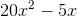Then, you can factor out the common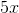to get your answer### Example Question #1 : How To Multiply Binomials With The Distributive Property

Which of the following expressions is equivalent to: 6x (m2 +yx2 3)?

6xm2 + 7x3 -18

xm2 + 7x3 -18

6xm2 + 6yx2 -18x

6xm2 + 6yx3 -18x

6xm2 + 6yx3 -18

6xm2 + 6yx3 -18x

Explanation:

6x (m2 +yx2 3)= 6x∙m2 + 6xyx2 – 6x∙3= 6xm2 + 6yx3 -18x (Use Distributive Property)

### Example Question #2 : How To Multiply Binomials With The Distributive Property

Which of the following expressions is equivalent to: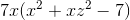?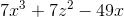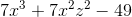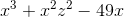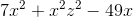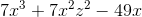Explanation:

Use the distributive property to multiply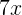by all of the terms in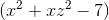: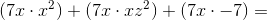### Example Question #3 : How To Multiply Binomials With The Distributive Property

Ifandare constants and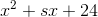is equivalent to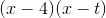, what is the value of?Cannot be determined from the given information.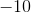Explanation:

The question gives us a quadratic expression and its factored form. From this, we know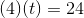At this point, solve for t.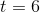Now, we can plug into get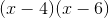.

Now, use FOIL to get s.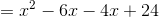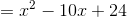### Example Question #4 : How To Multiply Binomials With The Distributive Property

Which expression is equal to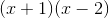?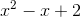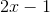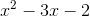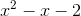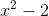Explanation:

In this problem, we are to multiply the two binomials using the FOIL method. This method stands for the order in which you multiply the variables. F stands for the first term of each binomial. O stands for the outside terms, meaning the first term of the first binomial and the last term of the second. I stands for the inside terms, meaning the second term of the first binomial and the first term of the second. L stands for the last term of each binomial. Once you do this, you simply add each part together to recieve your polynomial answer. Here is how our problem is solved: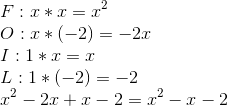### Example Question #5 : How To Multiply Binomials With The Distributive Property

Which expression is equal to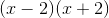?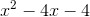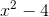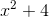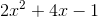Explanation:

In this problem, we are to multiply the two binomials using the FOIL method. This method stands for the order in which you multiply the variables. F stands for the first term of each binomial. O stands for the outside terms, meaning the first term of the first binomial and the last term of the second. I stands for the inside terms, meaning the second term of the first binomial and the first term of the second. L stands for the last term of each binomial. Once you do this, you simply add each part together to recieve your polynomial answer. Here is how our problem is solved: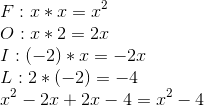### Example Question #6 : How To Multiply Binomials With The Distributive Property

Which expression is equal to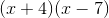?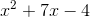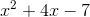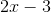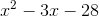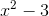Explanation:

In this problem, we are to multiply the two binomials using the FOIL method. This method stands for the order in which you multiply the variables. F stands for the first term of each binomial. O stands for the outside terms, meaning the first term of the first binomial and the last term of the second. I stands for the inside terms, meaning the second term of the first binomial and the first term of the second. L stands for the last term of each binomial. Once you do this, you simply add each part together to recieve your polynomial answer. Here is how our problem is solved: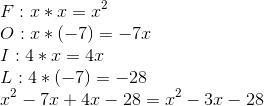### Example Question #7 : How To Multiply Binomials With The Distributive Property

Which expression is equal to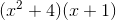?